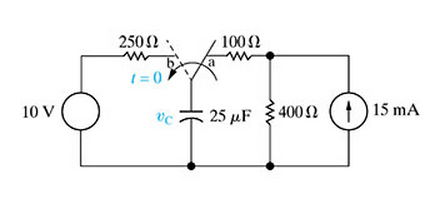Create an Account

Home / Questions / The switch in the circuit in Figure 1 has been in position a for a long time

The switch in the circuit in Figure 1 has been in position a for a long time

The switch in the circuit in (Figure 1) has been in position a for a long time. At t = 0, the switch moves instantaneously to position b and stays there.Find the initial value of the capacitor voltage.

Find the final value of the capacitor voltage.

Find the time constant for t ? 0.

Now suppose the switch in the circuit in the figure has been in position b for a long time. At t = 0, the switch moves instantaneously to position a and stays there. Find the initial value of the capacitor voltage.

Find the final value of the capacitor voltage.

Find the time constant for t?0.

Show transcribed image text The switch in the circuit in (Figure 1) has been in position a for a long time. At t = 0, the switch moves instantaneously to position b and stays there. Find the initial value of the capacitor voltage. Find the final value of the capacitor voltage. Find the time constant for t ? 0. Now suppose the switch in the circuit in the figure has been in position b for a long time. At t = 0, the switch moves instantaneously to position a and stays there. Find the initial value of the capacitor voltage. Find the final value of the capacitor voltage. Find the time constant for t?0.

Jul 27 2020 View more View LessSubscribe To Get Solution